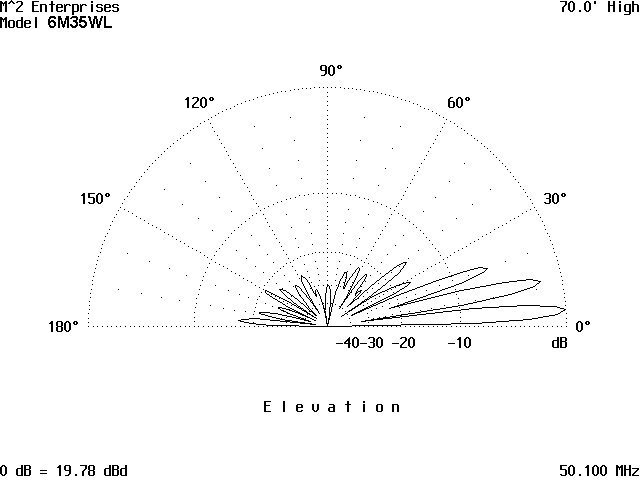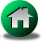## TYPICAL EFFECT OF ANTENNA HEIGHT ON GROUND GAIN LOBES

Below are comparisons of 6m yagis at 70' (3.5 wavelengths) and 150' (7.7 wavelengths) above the ground.  Note that the gain figures shown assume an average ground conductivity, which makes the forward gain figures (only when the antennas are pointed at the horizon) approximately 5.8 dB greater than the free space gain of the antennas (when they are pointed up in the sky).

At 70' height (3.5 wavelengths above ground):At 150' height (7.6 wavelengths above ground):Many thanks for VE7BQH for providing the above comparison charts.